# YOLO Explained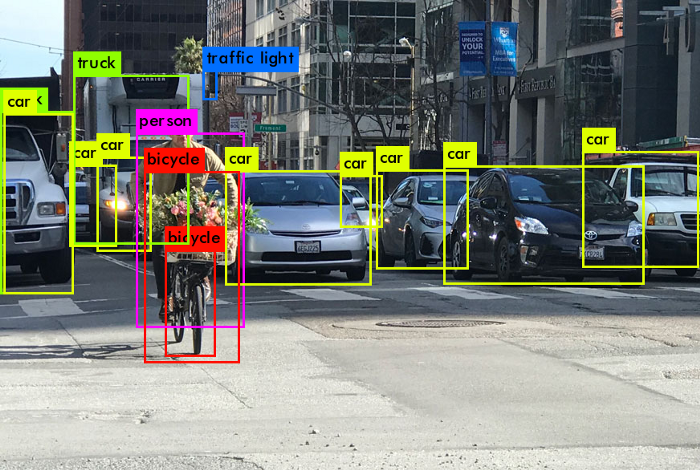Credit

# What is YOLO?

YOLO or You Only Look Once, is a popular real-time object detection algorithm. YOLO combines what was once a multi-step process, using a single neural network to perform both classification and prediction of bounding boxes for detected objects. As such, it is heavily optimized for detection performance and can run much faster than running two separate neural networks to detect and classify objects separately. It does this by repurposing traditional image classifiers to be used for the regression task of identifying bounding boxes for objects. This article will only look at YOLOv1, the first of the many iterations this architecture has gone through. Although the subsequent iterations feature numerous improvements, the basic idea behind the architecture stays the same. YOLOv1 referred to as just YOLO, can perform faster than real-time object detection at 45 frames per second, making it a great choice for applications that require real-time detection. It looks at the entire image at once, and only once — hence the name You Only Look Once — which allows it to capture the context of detected objects. This halves the number of false-positive detections it makes over R-CNNs which look at different parts of the image separately. Additionally, YOLO can generalize the representations of various objects, making it more applicable to a variety of new environments. Now that we have a general overview of YOLO, let’s take a look at how it really works.

# How Does YOLO Work?

YOLO is based on the idea of segmenting an image into smaller images. The image is split into a square grid of dimensions S×S, like so: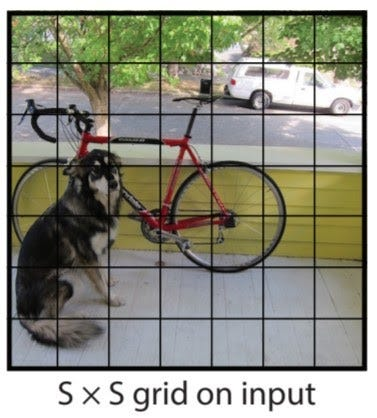Credit: research paper.

The cell in which the center of an object, for instance, the center of the dog, resides, is the cell responsible for detecting that object. Each cell will predict B bounding boxes and a confidence score for each box. The default for this architecture is for the model to predict two bounding boxes. The classification score will be from 0.0 to 1.0, with0.0 being the lowest confidence level and 1.0 being the highest; if no object exists in that cell, the confidence scores should be 0.0, and if the model is completely certain of its prediction, the score should be 1.0. These confidence levels capture the model’s certainty that there exists an object in that cell and that the bounding box is accurate. Each of these bounding boxes is made up of 5 numbers: the x position, the y position, the width, the height, and the confidence. The coordinates (x, y) represent the location of the center of the predicted bounding box, and the width and height are fractions relative to the entire image size. The confidence represents the IOU between the predicted bounding box and the actual bounding box, referred to as the ground truth box. The IOU stands for Intersection Over Union and is the area of the intersection of the predicted and ground truth boxes divided by the area of the union of the same predicted and ground truth boxes.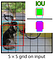Here is an example of an IOU: the area of intersection of the ground truth and predicted box in green divided by the area of the union of the two boxes, in purple. This will be between 0 and 1, 0 if they don’t overlap at all, and 1 if they are the same box. Therefore, a higher IOU is better as it is a more accurate prediction. Credit: image from research paper modified by me.

In addition to outputting bounding boxes and confidence scores, each cell predicts the class of the object. This class prediction is represented by a one-hot vector length C, the number of classes in the dataset. However, it is important to note that while each cell may predict any number of bounding boxes and confidence scores for those boxes, it only predicts one class. This is a limitation of the YOLO algorithm itself, and if there are multiple objects of different classes in one grid cell, the algorithm will fail to classify both correctly. Thus, each prediction from a grid cell will be of shape C + B * 5, where C is the number of classes and B is the number of predicted bounding boxes. B is multiplied by 5 here because it includes (x, y, w, h, confidence) for each box. Because there are S × S grid cells in each image, the overall prediction of the model is a tensor of shape S × S × (C + B ∗ 5).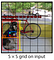Credit: image from research paper modified by me.

Here is an example of the output of the model when only predicting a single bounding box per cell. In this image, the dog’s true center is represented by the cyan circle labeled ‘object center’; as such, the grid cell responsible for detecting and bounding the box is the one containing the cyan dot, highlighted in dark blue. The bounding box that the cell predicts is made up of 4 elements. The red dot represents the center of the bounding box, (x, y), and the width and height are represented by the orange and yellow markers respectively. It is important to note that the model predicts the center of the bounding box with widths and heights rather than top left and bottom right corner positions. The classification is represented by a one-hot, and in this trivial example, there are 7 different classes. The 5th class is the prediction and we can see that the model is quite certain of its prediction. Keep in mind that this is merely an example to show the kind of output that is possible and so the values may not be accurate to any real values. Below is another image of all the bounding boxes and class predictions that would actually be made and their final result.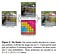Credit: image from research paper.

# YOLO Architecture

The YOLO model is made up of three key components: the head, neck, and backbone. The backbone is the part of the network made up of convolutional layers to detect key features of an image and process them. The backbone is first trained on a classification dataset, such as ImageNet, and typically trained at a lower resolution than the final detection model, as detection requires finer details than classification. The neck uses the features from the convolution layers in the backbone with fully connected layers to make predictions on probabilities and bounding box coordinates. The head is the final output layer of the network which can be interchanged with other layers with the same input shape for transfer learning. As discussed earlier, the head is an S × S × (C + B ∗ 5) tensor and is 7 × 7 × 30 in the original YOLO research paper with a split size S of 7, 20 classes C, and 2 predicted bounding boxes B. These three portions of the model work together to first extract key visual features from the image then classify and bound them.

# YOLO Training

As discussed previously, the backbone of the model is pre-trained on an image classification dataset. The original paper used the ImageNet 1000-class competition dataset and pre-trained 20 out of the 24 convolution layers followed by an average-pooling and fully connected layer. They then add 4 more convolutions to the model as well as 2 fully connected layers as it has been shown that adding both convulsions and fully connected layers increases performance. They also increased the resolution from 244 × 244 to 448 × 448 pixels as detection requires finer details. The final layer, which predicts both class probabilities and bounding box coordinates, uses a linear activation function while the other layers use a leaky ReLU function. The original paper trained for 135 epochs on the Pascal VOC 2007 and 2012 datasets using a batch size of 64. Data augmentation and dropout were used to prevent overfitting, with a dropout layer with a rate of 0.5, used between the first and second fully connected layers to encourage them to learn different things (preventing co-adaptation). There are more details available on the learning rate scheduling and other training hyperparameters in the original paper.

The loss function is the simple squared sum, but it must be modified. Without modification, the model will weight localization error, the difference between predicted and true bounding box coordinates, and class prediction error the same. Additionally, when a grid cell doesn’t contain an object, its confidence score tends towards 0 which can overpower the gradients from other cells that do contain objects. Both issues are solved by using two coefficients, λcoord and λnoobj, which multiply the loss for the coordinates and the object losses respectively. These are set to λcoord = 5 and λnoobj = 0.5, increasing the weight of detection and decreasing the importance of no object loss. Finally, to weight small bounding box equality as much as large boxes, the width and height difference is square-rooted rather than used directly. This makes sure that the error is treated the same as in large and small boxes, which would otherwise discourage the model from predicting large boxes. For example, if the predicted width of the bounding box is 10 and the actual width is 8, and we use this equation

we find the loss is 4. When we scale up to a predicted width of 100 and an actual of 98, the loss is 4 again. However, a difference of 2 out of the true 98 is negligible compared to a difference of 2 out of 8. Therefore, the loss between 10 and 8 should be much larger than the loss between 100 and 98. So we use this equation instead:

Using this new equation, the loss for 10 and 8 is 0.111 while the loss for 100 and 98 is 0.010. Keep in mind that looking at loss as a number by itself is meaningless, but the difference between values is meaningful. So the fact that 0.111 is much smaller than 4 doesn’t matter, but what does matter is that the difference between loss for the large and small widths is 0% for the squared difference while the difference is 90.99% for the squared rooted difference. This example shows why the square root is important: we want to treat big and small bounding boxes the same.

Each grid cell predicts multiple bounding boxes, but only one bounding box is responsible for detecting the object. The responsible bounding box is determined by choosing the predicted bounding box with the highest IOU. The effect of this is that certain bounding boxes will improve at predicting certain shapes and sizes of bounding boxes while others will specialize in other shapes. This occurs because of the following: if there is a large object when the multiple bounding boxes predict bounds, the best one is rewarded and continues to improve predicting large boxes. When a small object comes up, the previous predictor fails at predicting a good fit as its bounding box is too large. However, another predictor has a better prediction, and it is rewarded for bounding the small object well. As training goes on, the predictions of various bounding boxes diverge to specialize in the tasks they were good at early in training time.

Let’s look at the loss function:

Let’s break down the math.

The double summation merely means to sum across all of the grid cells (an S × S square) and all of the bounding boxes B.

This is an identity function, set to 1 if there is an object in cell i, and bounding box j is responsible for the prediction (it is responsible if it has the highest IOU, discussed earlier).

This represents the squared difference between the actual x coordinate and the predicted coordinate in cell i.

This is repeated for both x and y coordinates, finding the squared difference between the overall midpoint. Finally, the identity function is 0 when there is no object or the current bounding box isn’t the responsible one. In other words, we only calculate the loss for the best bounding box. So the first line of the equation is the sum of squared differences between the predicted and actual midpoints of the objects in all grid cells which have an object in them and are the responsible bounding box.

The second line is the sum of the squared differences between the square roots of the predicted and actual widths and heights in all grid cells which have an object in them. These are square rooted for reasons explained earlier.

The third line is just the squared difference between the predicted class probabilities and the actual class probabilities in all cells that contain an object.

The fourth line is the same but for all cells that don’t have an object in them. These two lines are summed across all bounding boxes because each bounding box also predicts a confidence score in addition to coordinates. The reason these two are split up is so that we can multiply the fourth line by the noobj coefficient to punish the model less severely if it misclassifies when there is no object present. There is one oddity though, as in line 4 we see the identity function for no object but it is not clear which bounding box is the responsible one to make the identity function a one. The research paper says the responsible box is the one with the highest IOU, but if there is no object there is no ground truth box, and consequently, no IOU values. One could train all bounding boxes or just the worst or any other combination, but the original paper doesn’t specify which they did.

The last line is a bit tricky: the first summation goes through every grid cell which has an object in it. Then, for that single grid cell, the squared difference between the predicted class vector and the actual vector is found.

For example, if this YOLO model was trained on 5 classes, it would predict a vector like pred in each cell. If the ground truth was the true vector, the loss for that cell would be as shown.

Finally, the last line is the squared difference between the predicted and actual class for all cells that have an object in them, which is basically just checking how far off the classification was. This is calculated just across grid cells and not across each bounding box as each cell predicts only a single classification regardless of the number of bounding boxes it also predicts. Finally, all of these are summed together, and the first two lines are multiplied by a coordinate coefficient to weight them more heavily and line four is multiplied by a smaller no object coefficient to weight it less.

# Limitations of YOLO

YOLO can only predict a limited number of bounding boxes per grid cell, 2 in the original research paper. And though that number can be increased, only one class prediction can be made per cell, limiting the detections when multiple objects appear in a single grid cell. Thus, it struggles with bounding groups of small objects, such as flocks of birds, or multiple small objects of different classes.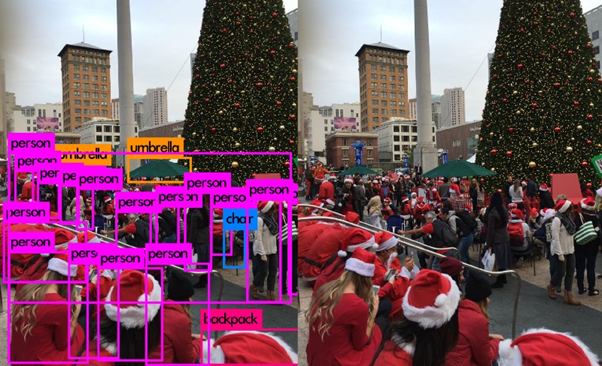Here you can see that only 5 people in the lower left-hand corner are detected by YOLO when there are at least 8 in the lower left-hand corner. Credit: Image source

# Conclusion

YOLO is an incredible computer vision model for object detection and classification. Hopefully, this article helped you understand how YOLO works at a high level. If you want to see the nitty-gritty details on a Python implementation, stick around: I will be publishing a follow-up blog on a PyTorch implementation of YOLO from scratch later, and following along with the code will be a great way to really test your understanding. And YOLO is only the first step in a larger project, a recurrent YOLO model which will further improve object detection and tracking across multiple frames, dubbed ROLO. Give me a follow to see the implementation of that, which will use recurrent networks in conjunction with YOLO. Thanks for reading, happy coding!

# Links

• The original research paper can be found as a pdf here
• More info on the research paper and their other publication is available on their site here
• All other sources are linked as they are used.

## Analytics Vidhya

Analytics Vidhya is a community of Analytics and Data…

## Sign up for Analytics Vidhya News Bytes

### By Analytics Vidhya

Latest news from Analytics Vidhya on our Hackathons and some of our best articles! Take a look.

By signing up, you will create a Medium account if you don’t already have one. Review our Privacy Policy for more information about our privacy practices.

Check your inbox
Medium sent you an email at to complete your subscription.

## Analytics Vidhya

Analytics Vidhya is a community of Analytics and Data Science professionals. We are building the next-gen data science ecosystem https://www.analyticsvidhya.com

Written by

## Ani Aggarwal

I am a high school student interested in AI and data science.## Analytics Vidhya

Analytics Vidhya is a community of Analytics and Data Science professionals. We are building the next-gen data science ecosystem https://www.analyticsvidhya.com

## More From Medium

Medium is an open platform where 170 million readers come to find insightful and dynamic thinking. Here, expert and undiscovered voices alike dive into the heart of any topic and bring new ideas to the surface. Learn more

Follow the writers, publications, and topics that matter to you, and you’ll see them on your homepage and in your inbox. Explore

If you have a story to tell, knowledge to share, or a perspective to offer — welcome home. It’s easy and free to post your thinking on any topic. Write on Medium